•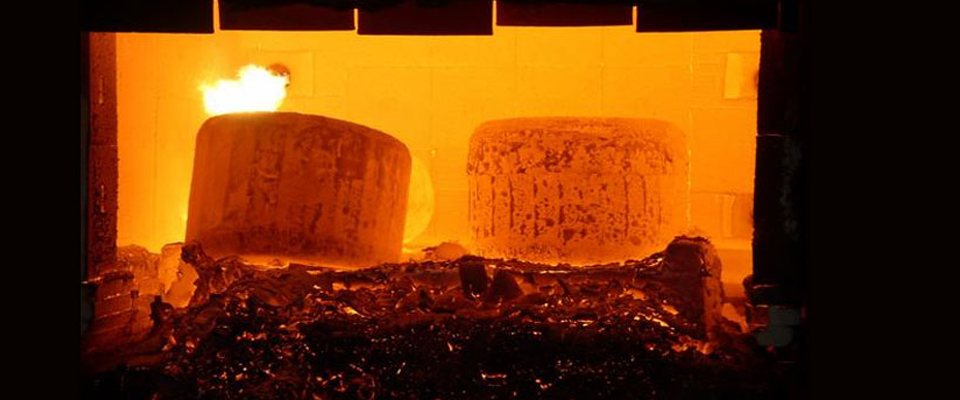••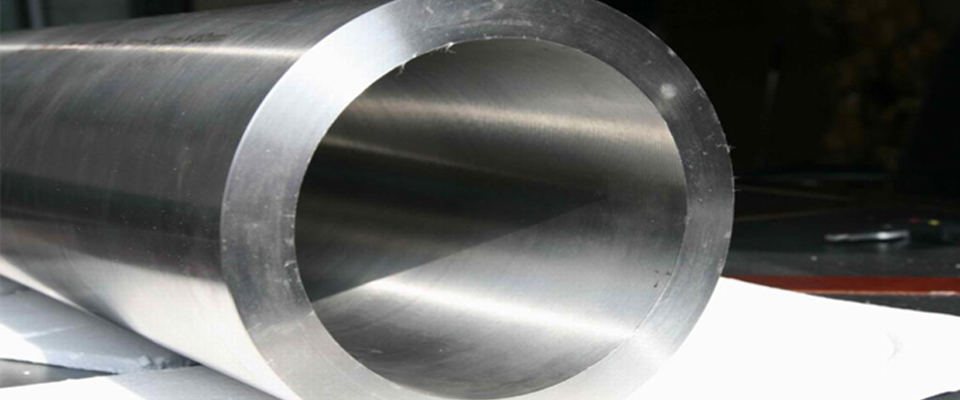•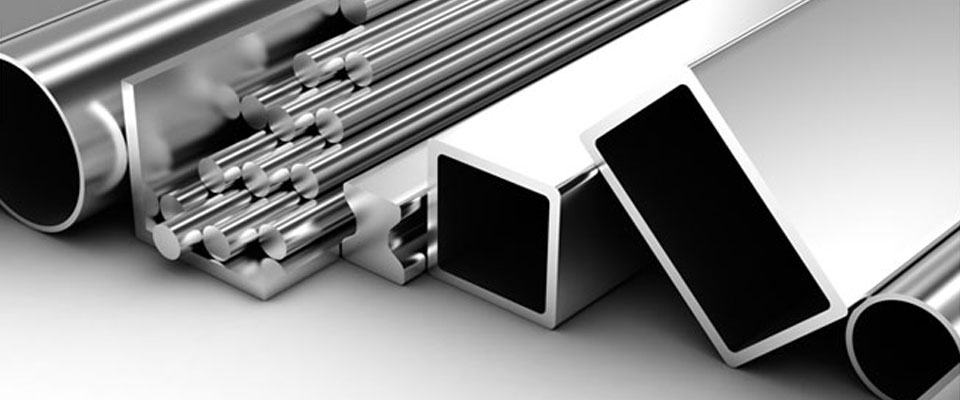•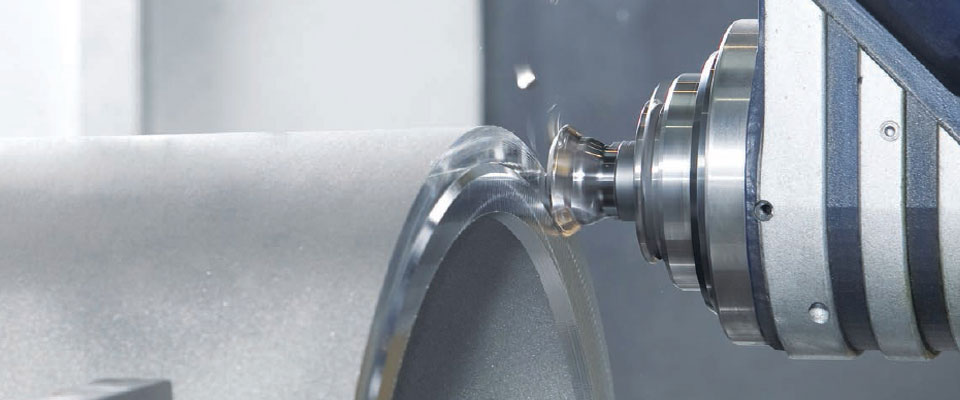# Theoretical Weight of Round Bar, Sheet or Plate

## Sheet Plate weight calculator, Round Bar weight calculator

### Sheet Plate weight formula, Round Bar weight formula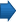Technical Information

Theoretical Weight of Round Bar, Sheet or Plate

Round Bar
The theoretical weight of a round bar can be
calculated using the following formula:

Weight = (Diameter² * Density Factor * 0.0007854 * Length) / 1000

Sheet or Plate
The theoretical weight of sheet or plate can be
calculated using the following formula:

Weight = (Length * Width * Density Factor * Thickness) / 1000000

Note: All measurements are in MM unit of measure.

 Grade Density Factor (KG / DM²) C276 8.89 22 8.69 200 8.89 201 8.89 400 8.83 K500 8.76 600 8.42 601 8.06 625 8.44 718 8.19 B2 9.22 B3 9.22 800 7.95 800H 7.95 800HT 7.95 F255 7.85

#### Theoretical Weight of Round Bar, Sheet or Plate, Sheet Plate weight calculator, Round Bar weight calculator, Sheet Plate weight formula, Round Bar weight formula

Technical Data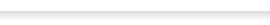Weight of Round Bar, Sheet or PlateFlanges DimensionsWerkstoff & UNS NumbersGrades and forms of Nickel Alloys and TitaniumConversions Tables

Products ListNickel AlloysNickel 200 / 201Monel 400Monel K500Inconel 600Inconel 601Inconel 617Inconel 625Inconel 718Incoloy 800 / H /HTIncoloy 825Hastelloy C276Hastelloy C22Hastelloy B2Hastelloy B3Hastelloy XTitanium AlloysAlloy 20904LDuplex & Super Duplex